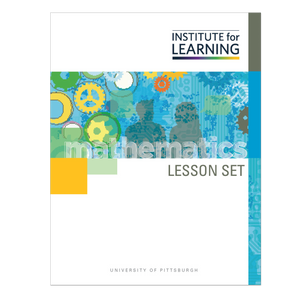# Locating, Ordering, and Finding Distance Between Positive and Negative Numbers (Grade 6)

Regular price \$6.25 \$0.00 Unit price per

In this lesson set, students learn strategies for locating and ordering positive and negative numbers, as well as strategies for determining the distance between positive and negative numbers. Through engaging in the tasks in this lesson set, students will:

• order positive and negative numbers on a number line;
• compare the relative size of positive and negative numbers; and
• calculate distance between positive and negative numbers.

Standards: 6.NS.C.5, 6.NS.C.6.A, 6.NS.C.6.C, 6.NS.C.7.A, 6.NS.C.7.C